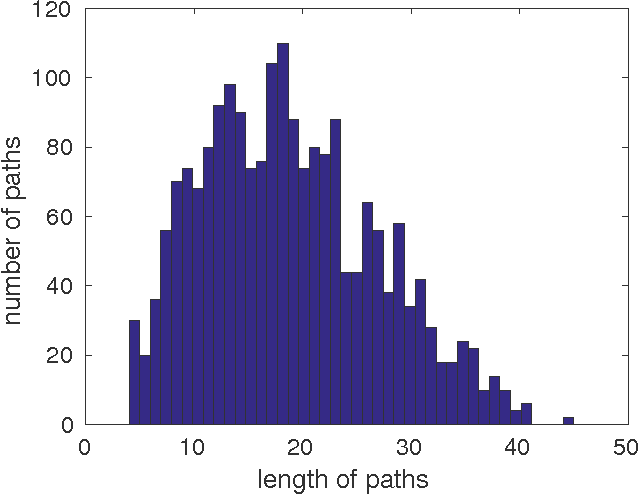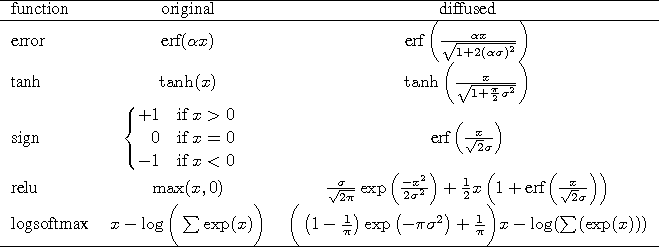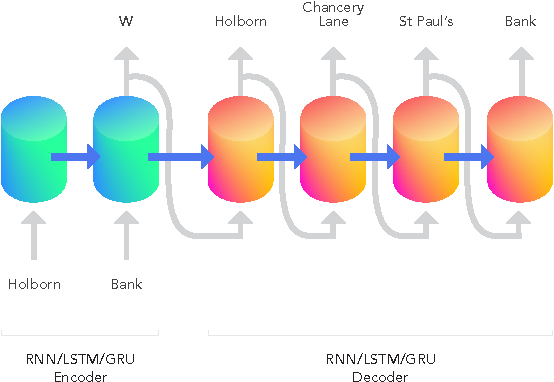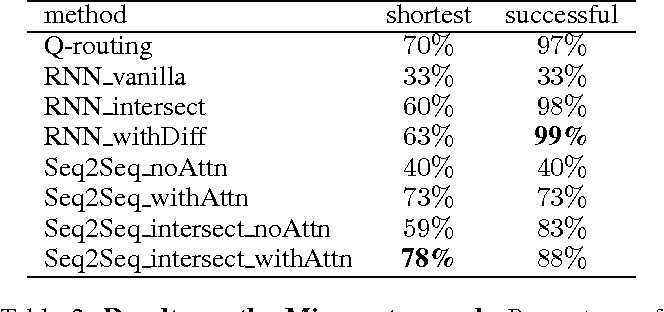Much combinatorial optimisation problems constitute a non-polynomial (NP) hard optimisation problem, i.e., they can not be solved in polynomial time. One such problem is finding the shortest route between two nodes on a graph. Meta-heuristic algorithms such as \$A^{*}\$ along with mixed-integer programming (MIP) methods are often employed for these problems. Our work demonstrates that it is possible to approximate solutions generated by a meta-heuristic algorithm using a deep recurrent neural network. We compare different methodologies based on reinforcement learning (RL) and recurrent neural networks (RNN) to gauge their respective quality of approximation. We show the viability of recurrent neural network solutions on a graph that has over 300 nodes and argue that a sequence-to-sequence network rather than other recurrent networks has improved approximation quality. Additionally, we argue that homotopy continuation -- that increases chances of hitting an extremum -- further improves the estimate generated by a vanilla RNN.Click To Get Code For Paper